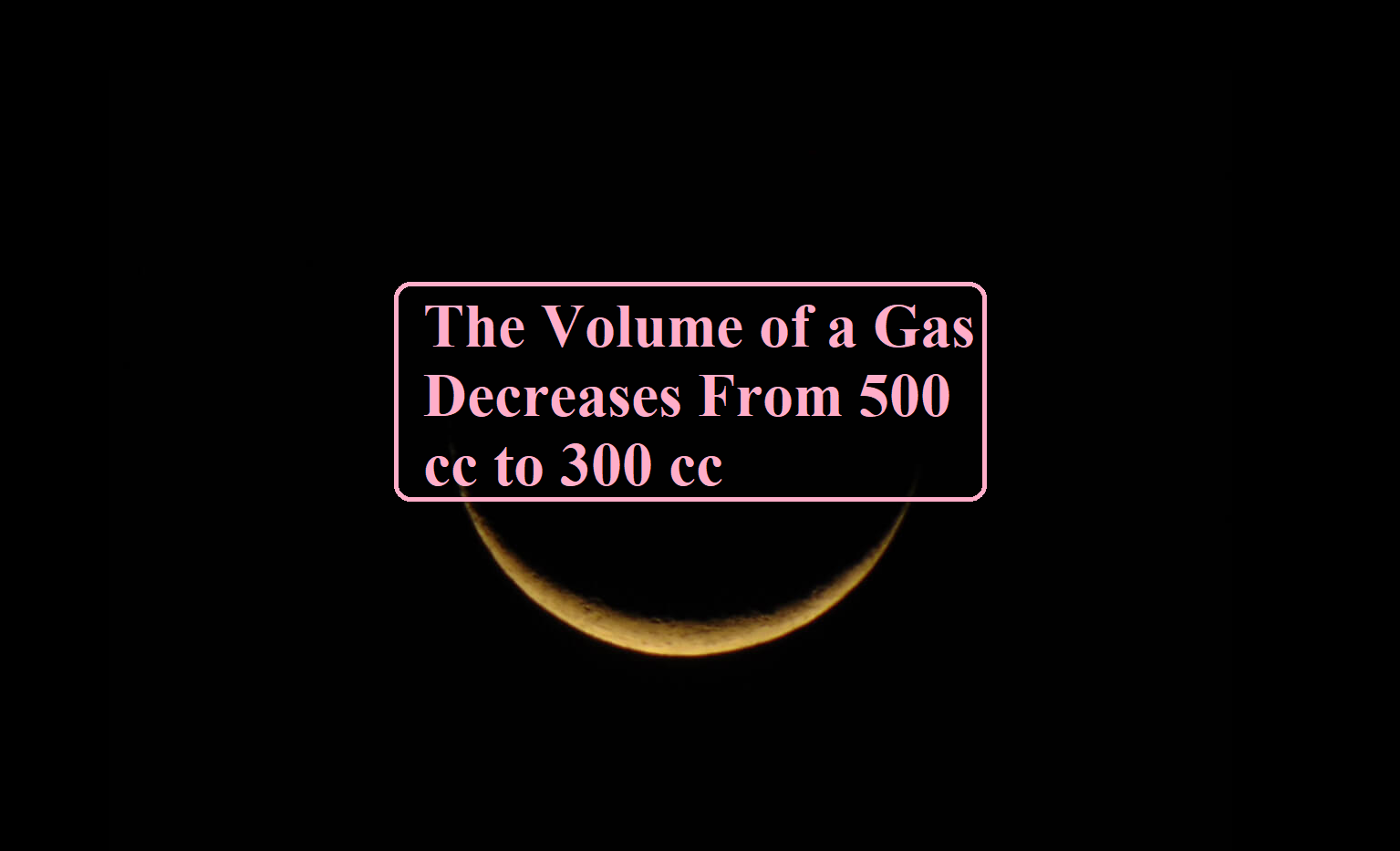# The Volume of a Gas Decreases From 500 cc to 300 ccThe Volume of a Gas Decreases From

Que. The volume of a gas decreases from 500 cc to 300 cc when a sample of gas is compressed by an average pressure of 0.6 atm. During this process, 10 J of heat is liberated. Find the change in internal energy

Ans: We know ΔE = q + W

Change in Volume (V) = 500 – 300 = 200 cc = 0.2 litre,

Pressure (P) = 0.6 atm and heat liberated (q) = 10 J

Work done (W) = PΔV = (0.2 × 0.6) = 0.12 litre – atm

But 1 litre – atm = 101.3 J.

Therefor W = 0.12 × 101.3 = 12.156 J.

We also know that heat is liberated, therefore it would be negative.

Thus, The change in ΔE = q + W = – 10 + 12.16 = 2.16 J.